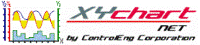XY Chart NET 3 Control Reference
ProfileXData Property

Use this property to get/set the x-data point values for a specific Profile.

Syntax
 [Visual Basic] Property ProfileXData(ProfileIndex As Integer) As Object [C#] object XYChartNETCtl.get_ProfileXData(int ProfileIndex) void  XYChartNETCtl.set_ProfileXData(int ProfileIndex, object Value) [C++] property System::Object ^ XYChartNet::XYChartNETCtl::ProfileXData[int]

Parameters
ProfileIndex
The index of the profile to set/get the x-data point values of.  Valid values are 0..(NumProfiles - 1).
Remarks

ProfileXData is a one-dimensional array (Number of Rows - 1) of double.  The number of rows is Profile(idx).NumSamples, where idx is the profile index to set/get the x-values for.

Initially, all data values are 0.  Two common methods to populate the ProfileXData array are:

1. Set ProfileXData equal to a one-dimensional array.
2. Read data from a CSV text file into a one-dimensional array, and then set ProfileXData equal to the array.
Example 1: Set ProfileXData equal to an array
```' XYChartNETCtl1 is the name of the XY Chart NET control instance placed on the form.

Dim arrData(4) As Double
Dim arrData2(8) As Double

arrData(0) = 0
arrData(1) = -0.1
arrData(2) = -0.2
arrData(3) = -0.3
arrData(4) = -0.4

arrData2(0) = 10
arrData2(1) = 20
arrData2(2) = 22
arrData2(3) = 33.5
arrData2(4) = 51
arrData2(5) = 65
arrData2(6) = 66
arrData2(7) = 68
arrData2(8) = 75

With XYChartNETCtl1
.NumProfiles = 2
.Profile(0).NumSamples = 5   '5 xy data points for Profile 0
.Profile(1).NumSamples = 9   '9 xy data points for Profile 1
.ProfileXData(0) = arrData
.ProfileXData(1) = arrData2
.Refresh                     'Refresh required to re-generate graph with new values
End With

'Get each profile's x-datapoints
arrData = XYChartNETCtl1.ProfileXData(0)
arrData2 = XYChartNETCtl1.ProfileXData(1)
```
```
```
```
```
Example 2: Read data from a CSV text file into an array, and then set ProfileXData equal to the array
```' XYChartNETCtl1 is the name of the XY Chart NET control instance placed on the form.

Dim FilePathAndName As String
Dim iFileNumber As Integer
Dim Row As Integer, Col As Integer
Dim DataArray() As Double

'Filepath below assumes source file is in the same folder as the application
FilePathAndName = App.Path + "\" + CSVFileName
iFileNumber = FreeFile()
Microsoft.VisualBasic.FileOpen(iFileNumber, FilePathAndName, OpenMode.Input)

With XYChartNETCtl1
.NumProfiles = 1
.Profile(0).NumSamples = 5   '5 xy data points for Profile 0

ReDim DataArray(.Profile(0).NumSamples - 1)

For Row = 0 To .Profile(0).NumSamples - 1
Microsoft.VisualBasic.Input(FileNumber, DataArray(Row))
Next Row

.ProfileXData(0) = DataArray
.Refresh                     'Refresh required to re-generate graph with new values
End With
Microsoft.VisualBasic.FileClose(iFileNumber)
```
```
```
```
```